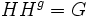Product of conjugates is proper

This article describes an easy-to-prove fact about basic notions in group theory, that is not very well-known or important in itself
View other elementary non-basic facts
VIEW FACTS USING THIS: directly | directly or indirectly, upto two steps | directly or indirectly, upto three steps|

This article describes a result of the form that argues that a subset constructed in a certain fashion is proper, viz it is not the whole group

Statement

Verbal statement

Given any two proper subgroups of a group that are conjugate to each other, their product is a proper subset of the group.

Symbolic statement

Let$H \le G$ be a proper subgroup, and let$H^g = g^{-1}Hg$ be a conjugate of$H$. Then$HH^g$ is a proper subset of$G$.

Proof

Given: A finite group$G$, two proper conjugate subgroups$H$ and$H^g$, where$H^g = g^{-1}Hg$.

To prove:$HH^g$ is a proper subset of$G$.

Proof: Suppose not, i.e., suppose$HH^g = G$.

1.$g \in HH^g$, so we can write$g = hk$ where$h \in H, k \in H^g$.
2. Thus,$H^g = H^{hk} = H^k$. This yields$H = (H^g)^{k^{-1}}$.
3. But we know that$k \in H^g$, so we get$H = H^g$.
4. We thus get$G = HH^g = H$, contradicting the assumption that$H$ is a proper subgroup of$G$.